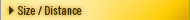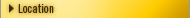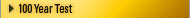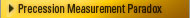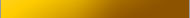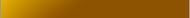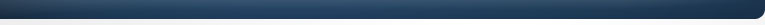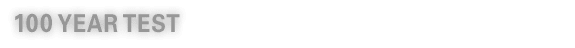In 1894 the Indian astronomer, Sri Yukteswar, wrote that the cause of the moving equinox (a.k.a. precession observable) was the result of our suns orbit around another star. He estimated the orbit period at 24,000 years and put apoapsis at about 500 A.D., saying the change in angular velocity (precession observable) would average 54 p/y over the life of the orbit. From Yukteswars orbit parameters, and utilizing the same rate of change numbers that Newcomb used for the period before 1900, we can apply Keplers laws to Yukteswars orbit data (constrained by a 24,000 year orbit period and apoapsis at 500A.D.) to come up with a forced eccentricity and expected rate of change for the 100 year period between 1900 and 2000 of .000349´´p/y.Yukteswar = .000349´´p/yNewcomb = .000222´´p/y Looking at Yukteswars orbit driven model of precession versus Newcombs local dynamics model of precession (both sets of data were given within a few years of each other over 100 years ago), we have an excellent long-term test of the two opposing models. The actual rate of change for the precession observable (or change in angular velocity traveled by the solar system along its binary orbit path in the dynamic SS model  seen as a change in orientation to the fixed stars) was .000346p/y. .000346 - .000349 = .000003´´ .000346 - .000222 = .000124´´ .000124 / .000003 = 41 Comparing Yukteswars and Newcombs predictions to the actual we find the dynamic SS model to be 41 times more accurate than the lunisolar precession model over the last 100 years. In this chart we have included precession calculations for 10 year periods, over the last 100 years.The first nine points are from Newcomb (or use Newcomb’s methodology) and the last three are from the Astronomical Almanac. The red line is a plot of the rate of increase (in arc length) required for a body in a 24,000 year orbit (Dynamic Solar System Model). Note the slope of the red line better fits the long term precession observable compared to Newcomb's calculations. It is a given (in the right column) as the annual rate of precession accelerates, the orbit period (or wobble cycle in lunisolor theory) will decrease. The ascending line is the plot one would expect to see if our sun were in a binary orbit with a total period of 24,000 years and mild eccentricity. Notice the historical data has a high correlation to our hypothesized orbit period. Lunisolar theory gives no good reason for the trend. A binary orbit, conforming to the laws of elliptical orbits, is a logical explanation. Change in arc length travelled p/y by a body in 24,000 year elliptical orbit from 1700 AD to 2100 ADChange in arc length traveled p/y by a body in a 24,000 year elliptical orbit from apoapsis, 500 AD, to periapsis, 12,500 AD.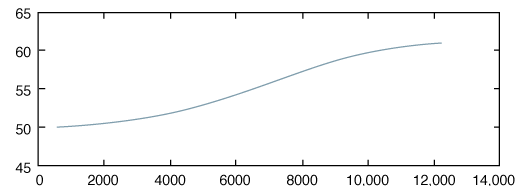Rate should range from a low of about 50 p/y to a high of about 62 p/y.